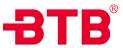• 系列号
T=T型角座阀
• 功能
7=常闭
8=常开
9=双作用
10=自由态
• 阀体材质
1=316L
2=316
3=304
• 执行器材质
5=304不锈钢
• 主要密封材质
9=PTFE(-20~+180℃)
1=PTFE复合(-20~+220℃)
• 执行器尺寸
03=32mm
• 接口
G=英制直管螺纹BSP DIN ISO228-1(国内G）